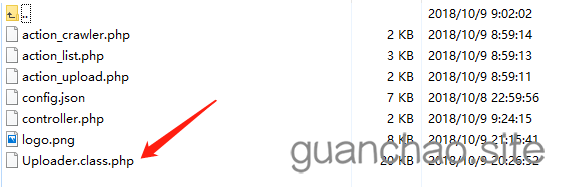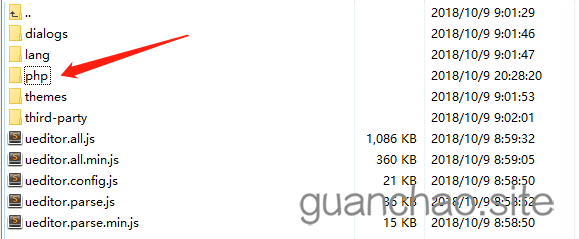# ueditor上傳圖片新增水印imageWaterMark是自定義的函式，``` php /     * 功能：PHP圖片水印 (水印支援圖片或文字)     * 引數：          \$groundImage 背景圖片，即需要加水印的圖片，暫只支援GIF,JPG,PNG格式；          \$waterPos水印位置，有10種狀態，0為隨機位置；          1為頂端居左，2為頂端居中，3為頂端居右；     4為中部居左，5為中部居中，6為中部居右；     7為底端居左，8為底端居中，9為底端居右；          \$waterImage圖片水印，即作為水印的圖片，暫只支援GIF,JPG,PNG格式；          \$waterText文字水印，即把文字作為為水印，支援ASCII碼，不支援中文；          \$textFont文字大小，值為1、2、3、4或5，預設為5；          \$textColor文字顏色，值為十六進位制顏色值，預設為#FF0000(紅色)；          * 注意：Support GD 2.0，Support FreeType、GIF Read、GIF Create、JPG 、PNG          \$waterImage 和 \$waterText 最好不要同時使用，選其中之一即可，優先使用 \$waterImage。          當\$waterImage有效時，引數\$waterString、\$stringFont、\$stringColor均不生效。          加水印後的圖片的檔名和 \$groundImage 一樣。     /     private function imageWaterMark(\$groundImage,\$waterPos=0,\$waterImage="",\$waterText="",\$textFont=5,\$textColor="#FF0000")     {         \$isWaterImage = FALSE;         \$formatMsg = "暫不支援該檔案格式，請用圖片處理軟體將圖片轉換為GIF、JPG、PNG格式。";         //讀取水印檔案         if(!empty(\$waterImage) && file_exists(\$waterImage))         {             \$isWaterImage = TRUE;             \$water_info = getimagesize(\$waterImage);             \$water_w = \$water_info;//取得水印圖片的寬             \$water_h = \$water_info;//取得水印圖片的高              switch(\$water_info)//取得水印圖片的格式             {                 case 1:\$water_im = imagecreatefromgif(\$waterImage);break;                 case 2:\$water_im = imagecreatefromjpeg(\$waterImage);break;                 case 3:\$water_im = imagecreatefrompng(\$waterImage);break;                 default:die(\$formatMsg);             }         }         //讀取背景圖片         if(!empty(\$groundImage) && file_exists(\$groundImage))         {             \$ground_info = getimagesize(\$groundImage);             \$ground_w = \$ground_info;//取得背景圖片的寬             \$ground_h = \$ground_info;//取得背景圖片的高             switch(\$ground_info)//取得背景圖片的格式             {                 case 1:\$ground_im = imagecreatefromgif(\$groundImage);break;                 case 2:\$ground_im = imagecreatefromjpeg(\$groundImage);break;                 case 3:\$ground_im = imagecreatefrompng(\$groundImage);break;                 default:die(\$formatMsg);             }         }         else         {             die("需要加水印的圖片不存在！");         }         //水印位置         if(\$isWaterImage)//圖片水印         {             \$w = \$water_w;             \$h = \$water_h;             \$label = "圖片的";         }         else//文字水印         {             \$temp = imagettfbbox(ceil(\$textFont*5),0,"./cour.ttf",\$waterText);//取得使用 TrueType 字型的文字的範圍             \$w = \$temp - \$temp;             \$h = \$temp - \$temp;             unset(\$temp);             \$label = "文字區域";         }         if( (\$ground_w<\$w) || (\$ground_h<\$h) )         {             echo "需要加水印的圖片的長度或寬度比水印".\$label."還小，無法生成水印！";             return;         }         switch(\$waterPos)         {             case 0://隨機                 \$posX = rand(0,(\$ground_w - \$w));                 \$posY = rand(0,(\$ground_h - \$h));                 break;             case 1://1為頂端居左                 \$posX = 0;                 \$posY = 0;                 break;             case 2://2為頂端居中                 \$posX = (\$ground_w - \$w) / 2;                 \$posY = 0;                 break;             case 3://3為頂端居右                 \$posX = \$ground_w - \$w;                 \$posY = 0;                 break;             case 4://4為中部居左                 \$posX = 0;                 \$posY = (\$ground_h - \$h) / 2;                 break;             case 5://5為中部居中                 \$posX = (\$ground_w - \$w) / 2;                 \$posY = (\$ground_h - \$h) / 2;                 break;             case 6://6為中部居右                 \$posX = \$ground_w - \$w;                 \$posY = (\$ground_h - \$h) / 2;                 break;             case 7://7為底端居左                 \$posX = 0;                 \$posY = \$ground_h - \$h;                 break;             case 8://8為底端居中                 \$posX = (\$ground_w - \$w) / 2;                 \$posY = \$ground_h - \$h;                 break;             case 9://9為底端居右                 \$posX = \$ground_w - \$w - 10;   // -10 是距離右側10px 可以自己調節                 \$posY = \$ground_h - \$h - 10;   // -10 是距離底部10px 可以自己調節                 break;             default://隨機                 \$posX = rand(0,(\$ground_w - \$w));                 \$posY = rand(0,(\$ground_h - \$h));                 break;         }         //設定影象的混色模式         imagealphablending(\$ground_im, true);         if(\$isWaterImage)//圖片水印         {             imagecopy(\$ground_im, \$water_im, \$posX, \$posY, 0, 0, \$water_w,\$water_h);//拷貝水印到目標檔案          }         else//文字水印         {             if( !emptyempty(\$textColor) && (strlen(\$textColor)==7) )             {                 \$R = hexdec(substr(\$textColor,1,2));                 \$G = hexdec(substr(\$textColor,3,2));                 \$B = hexdec(substr(\$textColor,5));             }             else             {                 die("水印文字顏色格式不正確！");             }             imagestring ( \$ground_im, \$textFont, \$posX, \$posY, \$waterText, imagecolorallocate(\$ground_im, \$R, \$G, \$B));          }         //生成水印後的圖片         @unlink(\$groundImage);         switch(\$ground_info)//取得背景圖片的格式         {             case 1:imagegif(\$ground_im,\$groundImage);break;             case 2:imagejpeg(\$ground_im,\$groundImage);break;             case 3:imagepng(\$ground_im,\$groundImage);break;             default:die(\$errorMsg);         }         //釋放記憶體         if(isset(\$water_info)) unset(\$water_info);         if(isset(\$water_im)) imagedestroy(\$water_im);         unset(\$ground_info);         imagedestroy(\$ground_im);     }

```